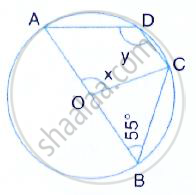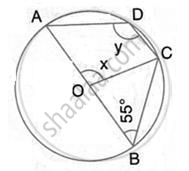Share

In the Given Figure, O is the Centre of the Circle and ∠Abc = 55°. Calculate the Values of X and Y. - ICSE Class 10 - Mathematics

ConceptArc and Chord Properties - the Angle that an Arc of a Circle Subtends at the Center is Double that Which It Subtends at Any Point on the Remaining Part of the Circle

Question

In the given figure, O is the centre of the circle and ∠ABC = 55°. Calculate the values of x and y.Solution∠AOC = 2 ∠ABC = 2 × 55°
(Angle at the centre is double the angle at the circumference subtended by the same chord)
∴ x = 110°
ABCD is cyclic quadrilateral
∴ ∠ADC +∠ ABC = 180°
(pair of opposite angles in a cyclic quadrilateral are supplementary
⇒  y = 180° - 55° = 125°

Is there an error in this question or solution?

Video TutorialsVIEW ALL 

Solution In the Given Figure, O is the Centre of the Circle and ∠Abc = 55°. Calculate the Values of X and Y. Concept: Arc and Chord Properties - the Angle that an Arc of a Circle Subtends at the Center is Double that Which It Subtends at Any Point on the Remaining Part of the Circle.
S